Windows

# State Details Window

This window shows the Hamiltonian matrix and the eigenvalues for a particular state (upper or lower), J and symmetry.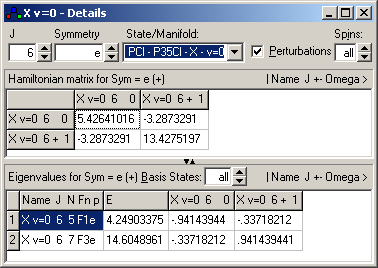## Selecting the State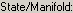Selects a state or manifold, (for a particular isotope, if applicable).Required total angular momentum, (or M if a static field is present).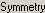Required symmetry - valid values depend on molecular point group.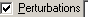Uncheck this box to exclude perturbations from the calculation.Set how many nuclear and electron spins to exclude from the calculation.

## The Hamiltonian Matrix

This shows the Hamiltonian Matrix before diagonalisation.Note the basis states in the row and column headings, and the key to the basis state quantum numbers on the right.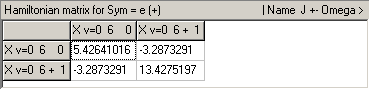## The Energy Levels and Eigenvectors

This shows the energy levels (second white column), the quantum numbers for the levels (first column, with the key to the quantum numbers on the top right) and the corresponding coefficients (subsequent columns). The columns are labeled by basis state, as in the Hamiltonian matrix.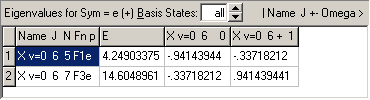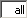If this is set to a number, n, then it shows the n largest coefficients in the eigenvector.